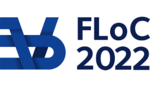FLOC 2022: FEDERATED LOGIC CONFERENCE 2022
TALK KEYWORD INDEX

A
algebraic complexity
algebraic proof systems
automatizability of proof systems
Average-case complexity
B
bit pigeonhole principle
blocked clauses
Bounded arithmetic
C
CDCL
constraint satisfaction problems
D
disjointness
E
elementary computation
enumeration
extended resolution
H
higher-order
hitting formulas
I
Invited Talk
K
knapsack
L
lambda calculus
lower bounds
M
multiparty communication complexity
N
Non-optimality of proof systems
O
optimal proof systems
P
perfect matching
polynomial calculus
preprocessing
proof complexity
propositional proof systems
Q
QBF
R
randomized communication complexity
resolution
resolution complexity
Resolution over linear equations
roots of unity
S
SAT encodings
separations
solvers
strategy extraction
sum-of-squares
symmetries
T
TBA
tree-like proofs
type theory